# Step by Step Guide for Long Division

## What is long division?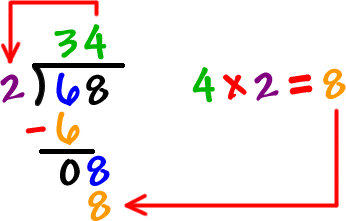Long division is a way to solve division problems with large numbers. Basically, these are division problems you cannot do in your head.

## Getting started

One of the problems students have with long division problems is remembering all the steps. Here’s a trick to mastering long division. Use the acronym DMSB, which stands for:

D = Divide
M = Multiply
S = Subtract
B = Bring down

This sequence of letters can be hard to remember, so think of the acronym in the context of a family:

Write D M S B in the corner of your worksheet to remember the sequence you’re about to use.

## How to write it down

First, you have to write down the problem in long division format. A typical division problem looks like this:

Dividend ÷ Divisor = Quotient

To write this down in long division format it looks like this:Let’s try a fairly simple example:

65 ÷ 5 = ?

Now, let’s write that problem down in the long division format: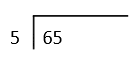We’re ready to start using the acronym: D M S B

## Step 1: D for Divide

How many times will 5 go into 65? That’s too hard to work out in your head, so let’s break it down into smaller steps.

The first problem you’ll work out in this equation is how many times can you divide 5 into 6. The answer is 1. So you put 1 on the quotient line.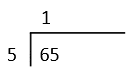## Step 2: M for Multiply

You multiply your answer from step 1 and your divisor: 1 x 5 = 5. You write 5 under the 6.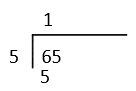## Step 3: S for Subtract

Next you subtract. In this case it will be 6 – 5 = 1.## Step 4: B for Bring down

The last step in the sequence is to bring down the next number from the dividend, which in this case is 5. You write the 5 next to the 1, making the number 15.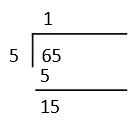Now you start all over again:

## Step 1: D for Divide

How many times can you divide 5 into 15. The answer is 3. So you put 3 on the quotient line.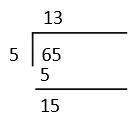## Step 2: M for Multiply

You multiply your answer from step 1 and your divisor: 3 x 5 = 15. Write this underneath the 15.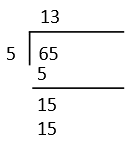## Step 3: S for Subtract

Now we subtract 15 from 15. 15 – 15 = 0.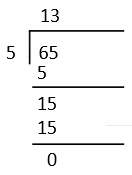There is no need for step 4. We have finished the problem.

Once you have the answer, do the problem in reverse using multiplication (5 x 13 = 65) to make sure your answer is correct.

K5 Learning has a number of free long division worksheets for grade 4, grade 5 and grade 6. Check them out in our math worksheet center.Become a Member

This content is available to members only.